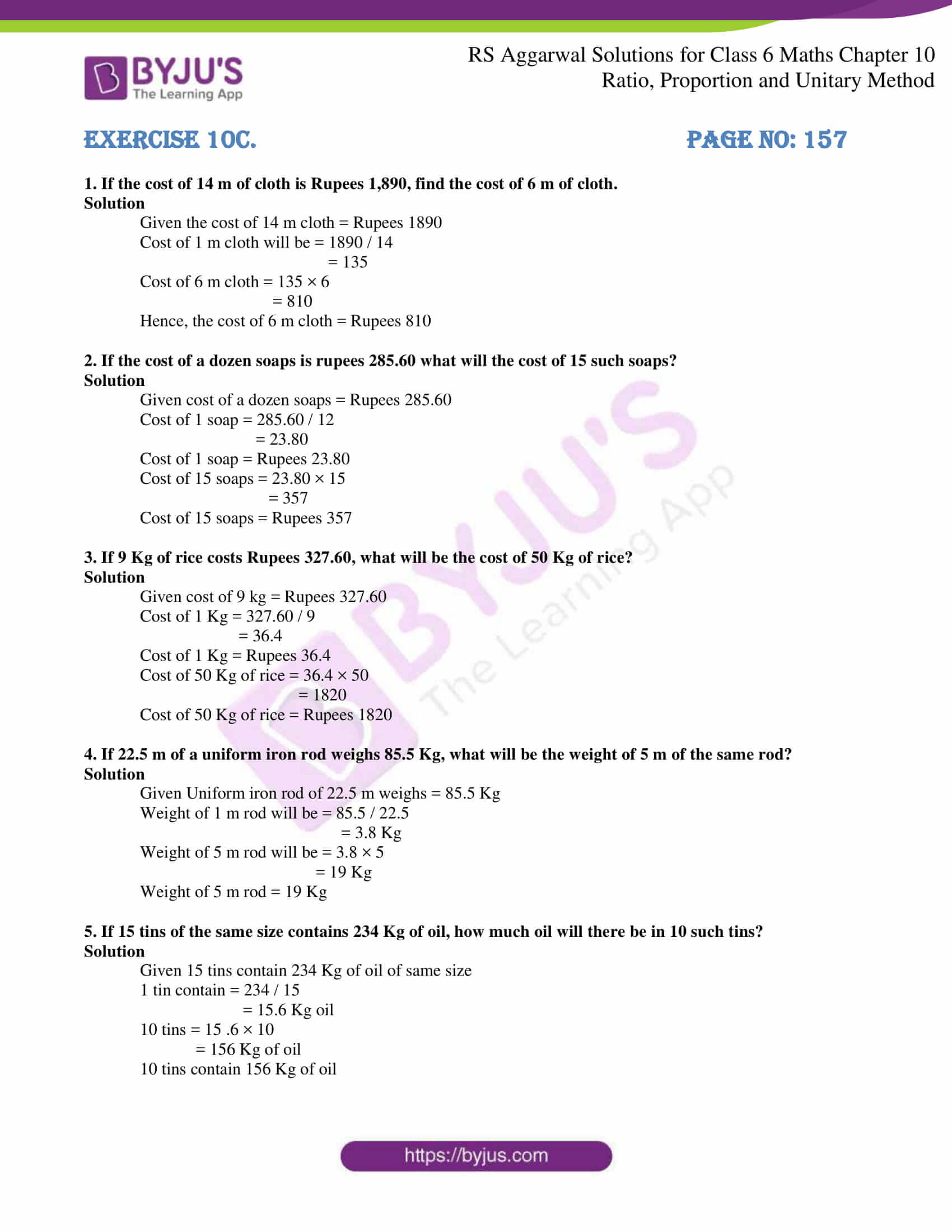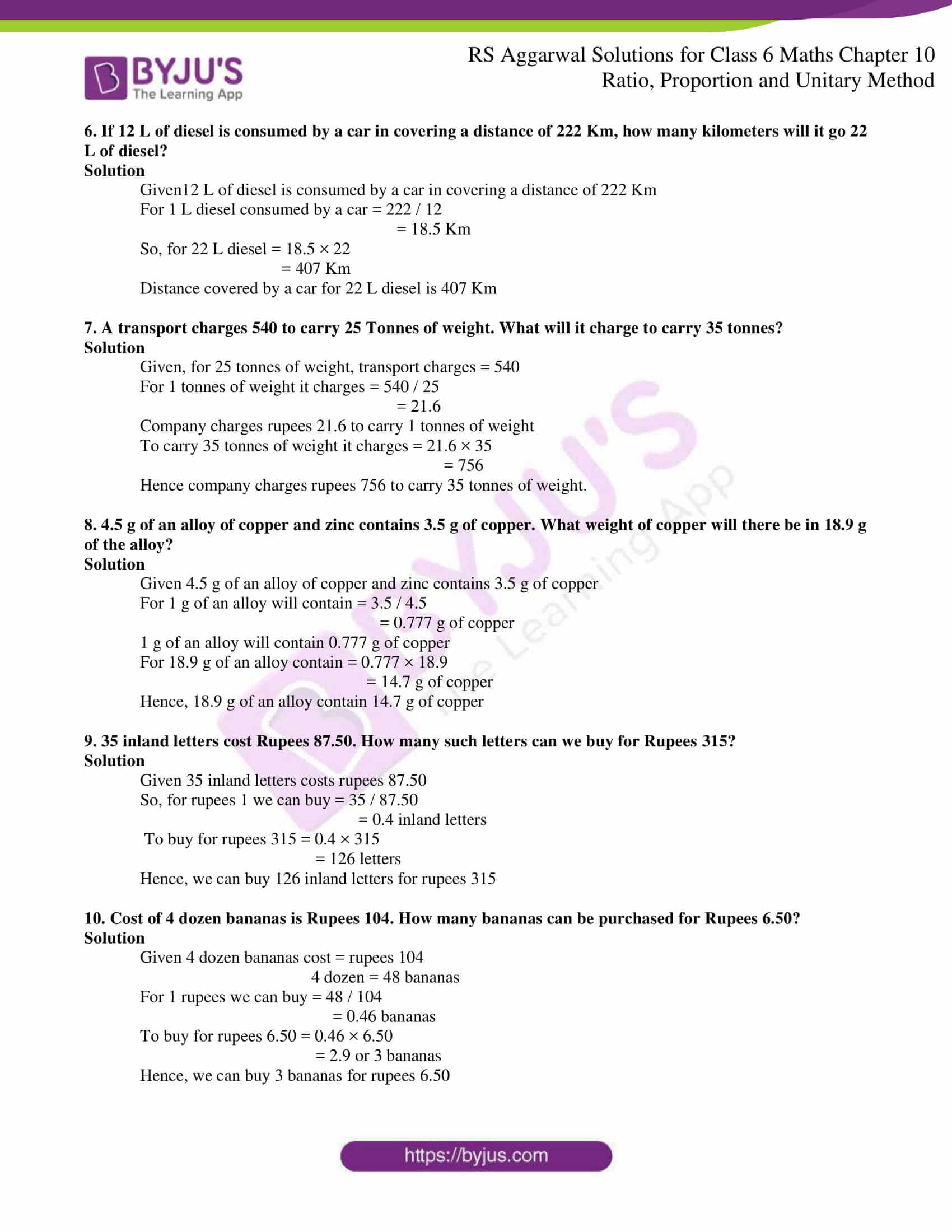# RS Aggarwal Solutions for Class 6 Chapter 10 Ratio, Proportion and Unitary Method Exercise 10C

This exercise mainly explains the students about the concept of the Unitary method. It is a method where we first find the value of one unit and then the value of the required number of units. The solutions are prepared in such a way they provide a better understanding of concepts among students. RS Aggarwal Solutions are very important for the students in order to perform better in the exam. In order to gain better knowledge about the topics explained here, the students can download RS Aggarwal Solutions for Class 6 Chapter 10 Ratio, Proportion and Unitary Method Exercise 10C PDF here.

## Download PDF of RS Aggarwal Solutions for Class 6 Chapter 10 Ratio, Proportion and Unitary Method Exercise 10C### Access answers to Maths RS Aggarwal Solutions for Class 6 Chapter 10 Ratio, Proportion and Unitary Method Exercise 10C

1. If the cost of 14 m of cloth is Rupees 1,890, find the cost of 6 m of cloth.

Solution

Given the cost of 14 m cloth = Rupees 1890

Cost of 1 m cloth will be = 1890 / 14

= 135

Cost of 6 m cloth = 135 × 6

= 810

Hence, the cost of 6 m cloth = Rupees 810

2. If the cost of a dozen soaps is rupees 285.60 what will the cost of 15 such soaps?

Solution

Given cost of a dozen soaps = Rupees 285.60

Cost of 1 soap = 285.60 / 12

= 23.80

Cost of 1 soap = Rupees 23.80

Cost of 15 soaps = 23.80 × 15

= 357

Cost of 15 soaps = Rupees 357

3. If 9 Kg of rice costs Rupees 327.60, what will be the cost of 50 Kg of rice?

Solution

Given cost of 9 kg = Rupees 327.60

Cost of 1 Kg = 327.60 / 9

= 36.4

Cost of 1 Kg = Rupees 36.4

Cost of 50 Kg of rice = 36.4 × 50

= 1820

Cost of 50 Kg of rice = Rupees 1820

4. If 22.5 m of a uniform iron rod weighs 85.5 Kg, what will be the weight of 5 m of the same rod?

Solution

Given Uniform iron rod of 22.5 m weighs = 85.5 Kg

Weight of 1 m rod will be = 85.5 / 22.5

= 3.8 Kg

Weight of 5 m rod will be = 3.8 × 5

= 19 Kg

Weight of 5 m rod = 19 Kg

5. If 15 tins of the same size contains 234 Kg of oil, how much oil will there be in 10 such tins?

Solution

Given 15 tins contain 234 Kg of oil of same size

1 tin contain = 234 / 15

= 15.6 Kg oil

10 tins = 15 .6 × 10

= 156 Kg of oil

10 tins contain 156 Kg of oil

6. If 12 L of diesel is consumed by a car in covering a distance of 222 Km, how many kilometers will it go 22 L of diesel?

Solution

Given12 L of diesel is consumed by a car in covering a distance of 222 Km

For 1 L diesel consumed by a car = 222 / 12

= 18.5 Km

So, for 22 L diesel = 18.5 × 22

= 407 Km

Distance covered by a car for 22 L diesel is 407 Km

7. A transport charges 540 to carry 25 Tonnes of weight. What will it charge to carry 35 tonnes?

Solution

Given, for 25 tonnes of weight, transport charges = 540

For 1 tonnes of weight it charges = 540 / 25

= 21.6

Company charges rupees 21.6 to carry 1 tonnes of weight

To carry 35 tonnes of weight it charges = 21.6 × 35

= 756

Hence company charges rupees 756 to carry 35 tonnes of weight.

8. 4.5 g of an alloy of copper and zinc contains 3.5 g of copper. What weight of copper will there be in 18.9 g of the alloy?

Solution

Given 4.5 g of an alloy of copper and zinc contains 3.5 g of copper

For 1 g of an alloy will contain = 3.5 / 4.5

= 0.777 g of copper

1 g of an alloy will contain 0.777 g of copper

For 18.9 g of an alloy contain = 0.777 × 18.9

= 14.7 g of copper

Hence, 18.9 g of an alloy contain 14.7 g of copper

9. 35 inland letters cost Rupees 87.50. How many such letters can we buy for Rupees 315?

Solution

Given 35 inland letters costs rupees 87.50

So, for rupees 1 we can buy = 35 / 87.50

= 0.4 inland letters

To buy for rupees 315 = 0.4 × 315

= 126 letters

Hence, we can buy 126 inland letters for rupees 315

10. Cost of 4 dozen bananas is Rupees 104. How many bananas can be purchased for Rupees 6.50?

Solution

Given 4 dozen bananas cost = rupees 104

4 dozen = 48 bananas

For 1 rupees we can buy = 48 / 104

= 0.46 bananas

To buy for rupees 6.50 = 0.46 × 6.50

= 2.9 or 3 bananas

Hence, we can buy 3 bananas for rupees 6.50